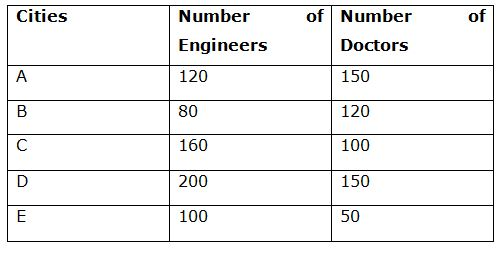# IBPS PO Prelims Quantitative Aptitude Questions 2019 (Day-3)

Dear Aspirants, Our IBPS Guide team is providing new series of Quantitative Aptitude Questions for IBPS PO Prelims 2019 so the aspirants can practice it on a daily basis. These questions are framed by our skilled experts after understanding your needs thoroughly. Aspirants can practice these new series questions daily to familiarize with the exact exam pattern and make your preparation effective.

Check here for IBPS PO Prelims Mock Test 2019

##### Check here for IBPS PO  Mock Test 2019
[WpProQuiz 6896]

Directions (1 – 5): Study the following information carefully and answer the given questions.

The table shows the number of engineers and doctors in five different cities viz, A, B, C, D and E.1) What is the ratio of the total number of engineers in city A, C and E together to that of the total number of doctors in city B, C and D together?

a) 37: 39

b) 31: 37

c) 38: 37

d) 41: 37

e) None of these

2) If the number of male engineers in city B is 26 and total number of males in city B is 48% of the total number of engineers in city D. What percentage of female doctors in city B from the total people (Engineers and Doctors)?

a) 15%

b) 20%

c) 24%

d) 25%

e) 28%

3) What is the difference between the number of doctors in city D and C is what percentage more/less than the number of engineers in city E?

a) 40%

b) 50%

c) 60%

d) 80%

e) 25%

4) What is the average number of Engineers in all the given cities together?

a) 139

b) 151

c) 129

d) 143

e) None of these

5) What is the ratio of the difference between the doctors in the city D and B to that of the difference between the engineers in the city A and C?

a) 2: 3

b) 5: 6

c) 8: 9

d) 3: 4

e) 7: 5

6) Ratio of the cost price to marked price of the article is 4: 5. A shopkeeper offer a discount of 15% and the marked price of the article is Rs.720. What is the profit percentage of the article?

a) 6.25%

b) 4.75%

c) 5.5%

d) 3.40%

e) None of these

7) A and B together can complete a work in 30 days. If B alone complete 20% of the work in 16 days. In how many days A alone complete 75% of the work?

a) 40 days

b) 36 days

c) 32 days

d) 28 days

e) None of these

8) A and B invests in the ratio of 2: 3 and the investment period of x months and y months respectively. If the total profit of the business is 3500 and B’s share is Rs.1500, then what is the ratio of x: y?

a) 2: 1

b) 3: 4

c) 4: 5

d) 1: 3

e) None of these

9) Shon invests Rs.8000 in scheme A which offer simple interest at 15% per annum for x years. After x years Shon received the total amount is Rs.17600. Find the value of x

a) 6 years

b) 7 years

c) 8 years

d) 10 years

e) None of these

10) Ratio of the speed of boat in still water to stream is 4: 1. If the boat rowing the speed of 20 kmph in still water, then what is the ratio of the speed of boat rowing against the current and along the current?

a) 2: 5

b) 3: 2

c) 3: 5

d) 5: 4

e) None of these

Required ratio = (120 + 160 + 100): (120 + 100 + 150)

= 380: 370 = 38: 37

Total number of male in city B = 48/100 * (200)

= 96

Number of male Doctors in city B = 96 – 26 = 70

Number of female doctors in city B = 120 – 70 = 50

Required percentage = 50/200 * 100 = 25%

Difference between doctors in city D and C = 150 – 100 = 50

Required percentage = (100 – 50)/100 * 100

= 50%

Required average = (120 + 80 + 160 + 200 + 100)/5

= 660/5 = 132

Required ratio = (150 – 120): (160 – 120)

= 30: 40 = 3: 4

Direction (6-10) :

CP = 4/5 * 720 = Rs.576

SP = 720 * 85/100 = Rs.612

Profit percentage = (612 – 576)/576 * 100

= 6.25%

A + B = 1/30

B = 5/1 * 16 = 80 days

A = 1/30 – 1/80

A = (8 – 3)/240 = 5/240

A = 1/48

A complete the 75% of the work in = 48 * ¾ = 36 days

A’s profit = 3500 – 1500 = 2000

(2 * x)/(3 * y) = 2000/1500

2x/3y = 4/3

x/y = 2/1

SI = (P * N * R)/100

SI = 17600 – 8000 = Rs.9600

9600 = (8000 * 15 * N)/100

N = 8 years

Speed of stream = ¼ * 20 = 5 kmph

Speed of the boat along the stream = 20 + 5 = 25 kmph

Speed of the boat against the stream = 20 – 5 = 15 kmph

Required ratio = 15: 25

= 3: 5